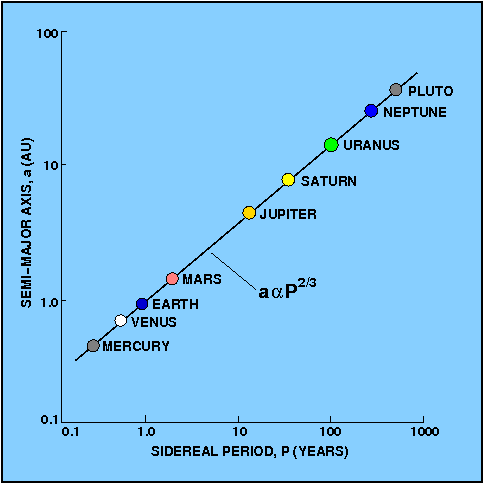Kepler worked for another decade looking for patterns in the motions of the planets. Finally, in 1619, he published his third law - the harmonic law:

The squares of the sidereal periods of the planets are proportional to the cubes of the semi-major axes of their orbits.

We have seen that the semi-major axis of a planet is equal to the mean distance of the planet, so an alternative form of Kepler's third law is that the cube of the mean distance of a planet is proportional to the square of its sidereal period.

 figure 33: Kepler's third law. Note that this figure is just a schematic and so should not be used to infer either the sidereal period or the semi-major axis of any of the planets.With his third law, Kepler had derived a relationship between the sizes of planetary orbits and their orbital periods. This relationship is shown in Figure 33 - a log-log plot of semi-major axis versus sidereal period for the planets of the solar system falls very close to a straight line of slope 2/3. Hence if a1 and T1 refer to the semi-major axis and sidereal period of a planet P1 moving about the Sun,

a13 / T12 = constant,

the constant being the same for any of the planetary orbits. If a2, a3, etc., and T2, T3, etc., refer to the semi-major axes and sidereal periods of the other planets P2, P3, etc., moving about the Sun, then

a13 / T12 = a23 / T22 = a33 / T32 = constant.

The most convenient form of the constant is obtained by taking the planet to be the Earth and expressing the distance in astronomical units and the time in years. Hence, for the Earth, a = 1 and T = 1, so the constant becomes unity. For any other planet, consequently,

a3 = T2,

showing that if we measure the sidereal period, T, of a planet in Earth-years, we can obtain its mean distance from the Sun, a, in AU, as shown in the example problems.

By 1621, Kepler had shown that the four moons of Jupiter discovered by Galileo also obeyed his third law, but with a different value of the constant, confirming its wide applicability. As we shall see when we discuss Newton's derivation of Kepler's laws, however, the so-called constant in Kepler's third law is not really a constant but is dependent on the masses of the two celestial bodies in orbit about each other. Nevertheless, when one of the bodies is significantly more massive that the other, such as the Sun compared to the planets, or Jupiter compared to its satellites, Kepler's third law is a very close approximation to the truth. Only when the outer-most retrograde satellites in the solar system are considered, or close satellites of a non-spherical planet, do Kepler's laws fail to describe in their usual highly accurate manner the behaviour of such bodies. Even then, however, they may be used as a first approximation.